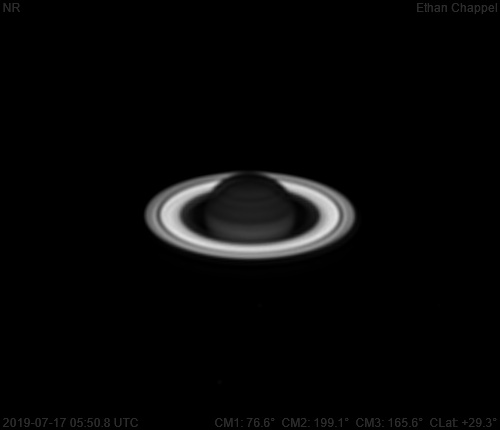# Saturn 2019-07-17 05:50 UTC

CM1: 76.60°

CM2: 199.10°

CM3: 165.60°

CLat: +29.30°

Description

Like most nights this summer, it was slightly too windy for the CGEM DX/C14. Other than that, the conditions for imaging were great.

Notes:

• Dione is near the four o'clock position relative to Saturn. Rhea, and Tethys are near the six o'clock position. Enceladus should be near Tethys, but doesn't seem to be have been bright enough to be detected.

Equipment

Celestron EdgeHD 8

Logs
```FireCapture v2.6  Settings
------------------------------------
Observer=Ethan Chappel
Camera=ZWO ASI290MM
Filter=CH4
Profile=Jupiter
Diameter=44.17"
Magnitude=-2.50
CMI=205.1° CMII=88.4° CMIII=68.4°  (during mid of capture)
FocalLength=1700mm (F/6)
Resolution=0.35"
Filename=2019-07-17-0501_1-EC-CH4-Jup.ser
Date=2019_07_17
Start=04_59_11.486
Mid=05_01_11.499
End=05_03_11.513
Start(UT)=04_59_11.486
Mid(UT)=05_01_11.499
End(UT)=05_03_11.513
Duration=240.027s
Date_format=yyyy_MM_dd
Time_format=HH_mm_ss
LT=UT -6h
Frames captured=3429
File type=SER
Binning=no
Bit depth=8bit
Debayer=no
ROI=252x212
ROI(Offset)=8x0
FPS (avg.)=14
Shutter=70.00ms
Gain=400 (66%)
USBTraffic=40 (off)
FPS=100 (off)
Brightness=1 (off)
AutoExposure=off
Gamma=50 (off)
SoftwareGain=10 (off)
AutoHisto=75 (off)
AutoGain=off
HighSpeed=off
Histogramm(min)=0
Histogramm(max)=220
Histogramm=86%
Noise(avg.deviation)=0.31
AutoAlign=false
PreFilter=none
Limit=240 Seconds
Sensor temperature=32.7°C
FireCapture v2.6  Settings
------------------------------------
Observer=Ethan Chappel
Camera=ZWO ASI290MM
Filter=CH4
Profile=Jupiter
Diameter=44.17"
Magnitude=-2.50
CMI=202.5° CMII=85.8° CMIII=65.8°  (during mid of capture)
FocalLength=1700mm (F/6)
Resolution=0.36"
Filename=2019-07-17-0456_9-EC-CH4-Jup.ser
Date=2019_07_17
Start=04_54_56.697
Mid=04_56_56.698
End=04_58_56.700
Start(UT)=04_54_56.697
Mid(UT)=04_56_56.698
End(UT)=04_58_56.700
Duration=240.003s
Date_format=yyyy_MM_dd
Time_format=HH_mm_ss
LT=UT -6h
Frames captured=3429
File type=SER
Binning=no
Bit depth=8bit
Debayer=no
ROI=252x212
ROI(Offset)=8x0
FPS (avg.)=14
Shutter=70.00ms
Gain=400 (66%)
USBTraffic=40 (off)
FPS=100 (off)
Brightness=1 (off)
AutoExposure=off
Gamma=50 (off)
SoftwareGain=10 (off)
AutoHisto=75 (off)
AutoGain=off
HighSpeed=off
Histogramm(min)=0
Histogramm(max)=194
Histogramm=76%
Noise(avg.deviation)=0.31
AutoAlign=false
PreFilter=none
Limit=240 Seconds
Sensor temperature=33.1°C
```